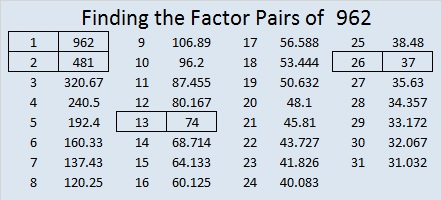# 962 Buckle Up, Pilgrim

Perhaps the most distinctive element of the pilgrims’ wardrobe is the buckle. We see it around the men’s waists, on their shoes, and on their hats. At least we do we if are looking at pilgrim costumes. It probably didn’t play as prominent a role on their actual clothes. Nevertheless, it is featured here on today’s puzzle. So buckle up and see where this puzzle takes you.Print the puzzles or type the solution in this excel file: 12 factors 959-967

Here are a few facts about the number 962:

It is the sum of two squares two different ways:
31² +  1² = 962
29² +  11² =  962

It is also the hypotenuse of four Pythagorean triples:
62-960-962, calculated from 2(31)(1), 31² –  1², 31² +  1²
312-910-962, which is 2 times (156-455-481)
370-888-962, which is 2 times (185-444-481)
638-720-962, calculated from 2(29)(11), 29² –  11², 29² +  11²

It looks interesting in a few other bases:
4242 BASE 6
282 BASE 20
101 BASE 31
QQ BASE 36 (Q is 26 in base 10)

• 962 is a composite number.
• Prime factorization: 962 = 2 × 13 × 37
• The exponents in the prime factorization are 1, 1, and 1. Adding one to each and multiplying we get (1 + 1)(1 + 1)(1 + 1) = 2 × 2 × 2 = 8. Therefore 962 has exactly 8 factors.
• Factors of 962: 1, 2, 13, 26, 37, 74, 481, 962
• Factor pairs: 962 = 1 × 962, 2 × 481, 13 × 74, or 26 × 37
• 962 has no square factors that allow its square root to be simplified. √962 ≈ 31.01612